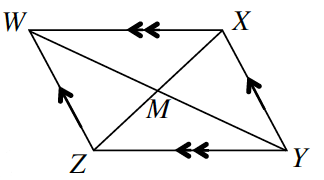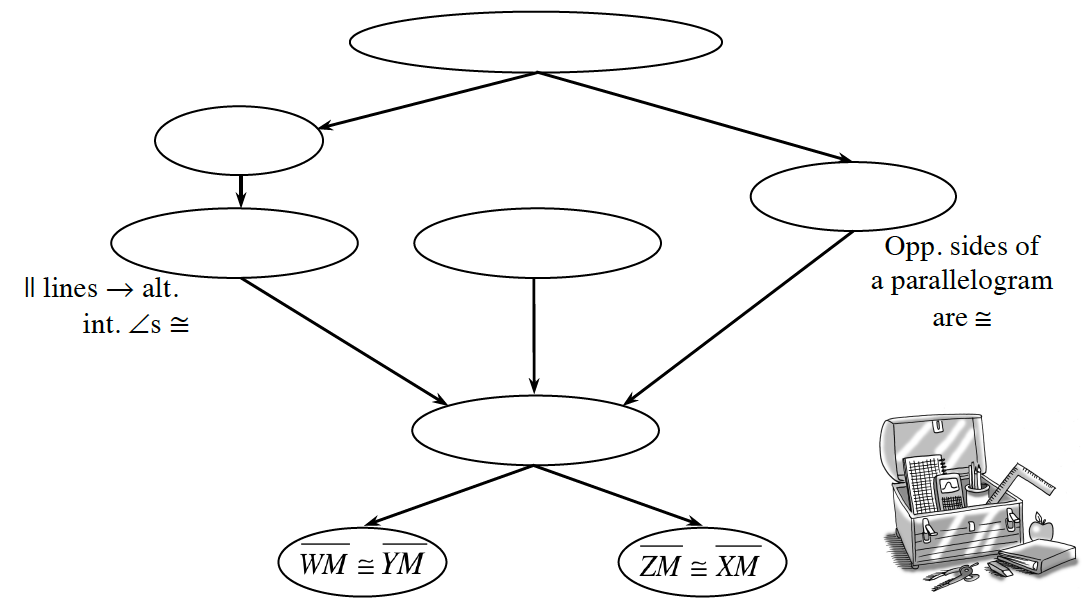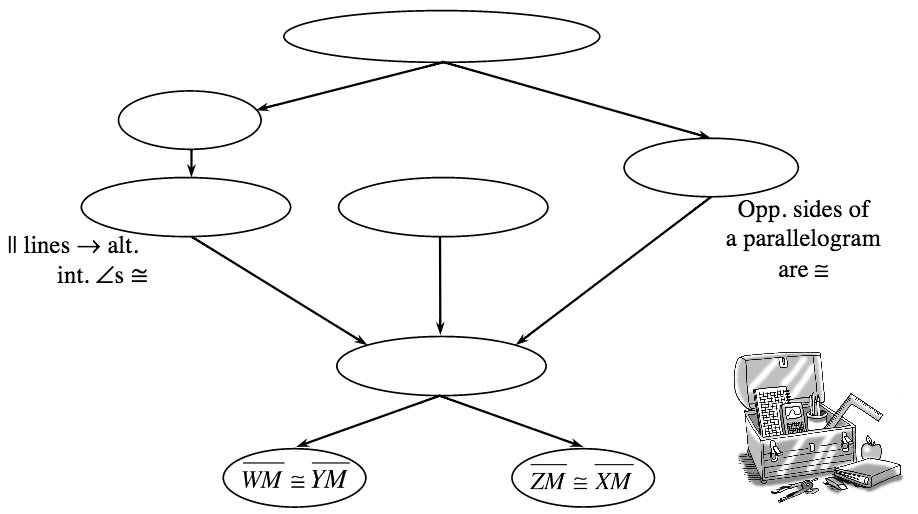### Home > INT2 > Chapter 7 > Lesson 7.1.1 > Problem7-10

7-10.

What else can you prove about parallelograms? Prove that the diagonals of a parallelogram bisect each other. For example, assuming that quadrilateral $WXYZ$ at right is a parallelogram, prove that $\overline{WM} ≅ \overline{YM}$ and $\overline{ZM} ≅ \overline{XM}$ . Fill in the missing statements and reasons in the flowchart proof below. Then record your theorem in your Theorem Graphic Organizer.Start by proving $ΔXWM ≅ ΔZYM$ by ASA$≅$. Think about parallel lines and angles while working on this proof.

What reason justifies that $\overline{WM} ≅ \overline{YM}$ and $\overline{ZM} ≅ \overline{XM}$ ?